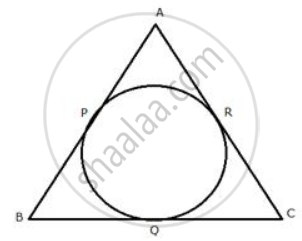Share

# In the Figure of Question 9; If Ab = Ac Then Prove that Bq = Cq. - Mathematics

Course

#### Question

In the following figure; If AB = AC then prove that BQ = CQ.#### Solution

Since, from A, AP and AR are the tangents to the circle
Therefore, AP = AR
Similarly, we can prove that

BP = BQ and CR = CQ
AP + BP + CQ = AR + BQ + CR
(AP + BP) + CQ = (AR + CR) + BQ
AB + CQ = AC + BQ
But AB = AC
Therefore, CQ = BQ or BQ = CQ

Is there an error in this question or solution?